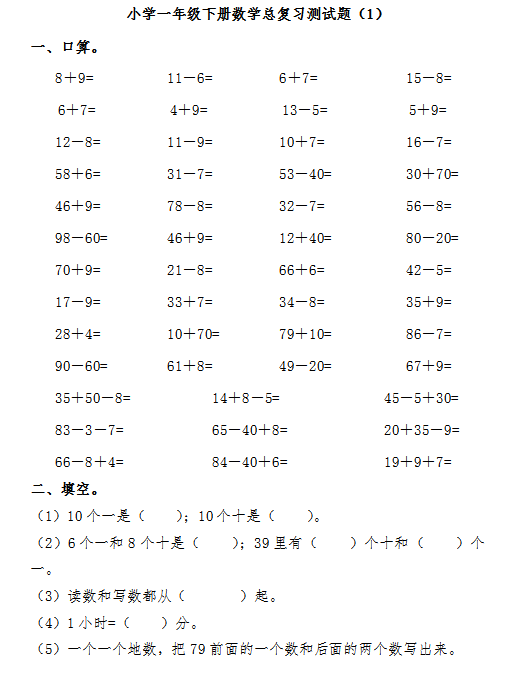8＋9=          11－6=         6＋7=            15－8=

6＋7=          4＋9=          13－5=           5＋9=

12－8=         11－9=         10＋7=           16－7=

58＋6=         31－7=         53－40=          30＋70=